# SSAR, College Fairs, FAFSA opens 10/01, UF Housing deposits & more!# Hello, The College Map!

 table div table+table+table+table div table{width:100%;padding:0}table div table+table+table+table div table img{width:96.23%;padding:0;float:none}table div table+table+table+table div table td{width:100%;padding:0 1.88% 18px}/* styles */## What is the SSAR?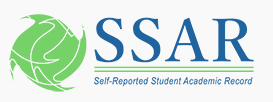table div table+table+table+table+table+table+table+table div table{width:100%;padding:0}table div table+table+table+table+table+table+table+table div table img{width:96.23%;padding:0;float:none}table div table+table+table+table+table+table+table+table div table td{width:100%;padding:0 1.88% 18px}/* styles */## FAFSA Opens on 10/01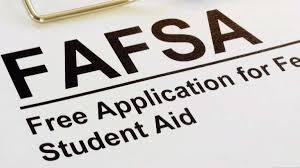Seniors! The FAFSA (Free Application for Federal Student Aid) opens on October 1st. If you are planning on attending a 4-year university, you must fill out the FAFSA. Most all scholarships require it. So, the FAFSA is a must!! Even if you believe your parents make too much money to qualify for need based aid, you must complete the application for merit or academic based scholarships! Attached are some videos below to help you navigate through the process. Go ahead and make your FSA ID’s then you’ll be ready for the FAFSA on October 1st.

FAFSA Help:

1. Create an FSA ID for you and (1) of your parents: www.studentaid.gov/fsaid That is your electronic signature and takes about 1-2 days to be confirmed with the Social Security Administration. Make sure you and one of your parents (legal guardian) have created an FSA ID. They cannot be the same username and email address. Attached is a FSA ID youtube video link below:
 1 Create an FSA ID for you and (1) of your parents: www.studentaid.gov/fsaid That is your electronic signature and takes about 1-2 days to be confirmed with the Social Security Administration. Make sure you and one of your parents (legal guardian) have created an FSA ID. They cannot be the same username and email address. Attached is a FSA ID youtube video link below:

 table div table+table+table+table+table+table+table+table+table+table+table+table div table{width:100%;padding:0}table div table+table+table+table+table+table+table+table+table+table+table+table div table img{width:96.23%;padding:0;float:none}table div table+table+table+table+table+table+table+table+table+table+table+table div table td{width:100%;padding:0 1.88% 18px}/* styles */## NACAC College Fair in Orlando!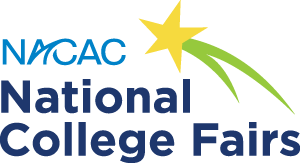/* styles */ Sunday, 10/5 from 1pm-4pm at the Orange County Convention Center! National College Fairs allow students to interact one-on-one with college admission representatives from a wide range of postsecondary institutions. Free and open to the public, NACAC fairs connect you with hundreds of colleges and universities in one location. There are several types of fairs to help you find your perfect fit! You can register HERE.
 table div table+table+table+table+table+table+table+table+table+table+table+table+table+table+table+table div table{width:100%;padding:0}table div table+table+table+table+table+table+table+table+table+table+table+table+table+table+table+table div table img{width:96.23%;padding:0;float:none}table div table+table+table+table+table+table+table+table+table+table+table+table+table+table+table+table div table td{width:100%;padding:0 1.88% 18px}/* styles */## Discover Duke University/* styles */ You and your family are invited to attend the DISCOVER DUKE program in Orlando this fall. Duke admissions officers will present information and answer questions about the university, the admissions process, financial aid, and much more. Program will be held on October 6th at 3pm: Springhill Suites by Marriott Orlando Airport 5828 Hazeltine National Drive Orlando, FL 32822 Please register here.
 table div table+table+table+table+table+table+table+table+table+table+table+table+table+table+table+table+table+table+table+table div table{width:100%;padding:0}table div table+table+table+table+table+table+table+table+table+table+table+table+table+table+table+table+table+table+table+table div table img{width:96.23%;padding:0;float:none}table div table+table+table+table+table+table+table+table+table+table+table+table+table+table+table+table+table+table+table+table div table td{width:100%;padding:0 1.88% 18px}/* styles */## University of Florida Housing Deposits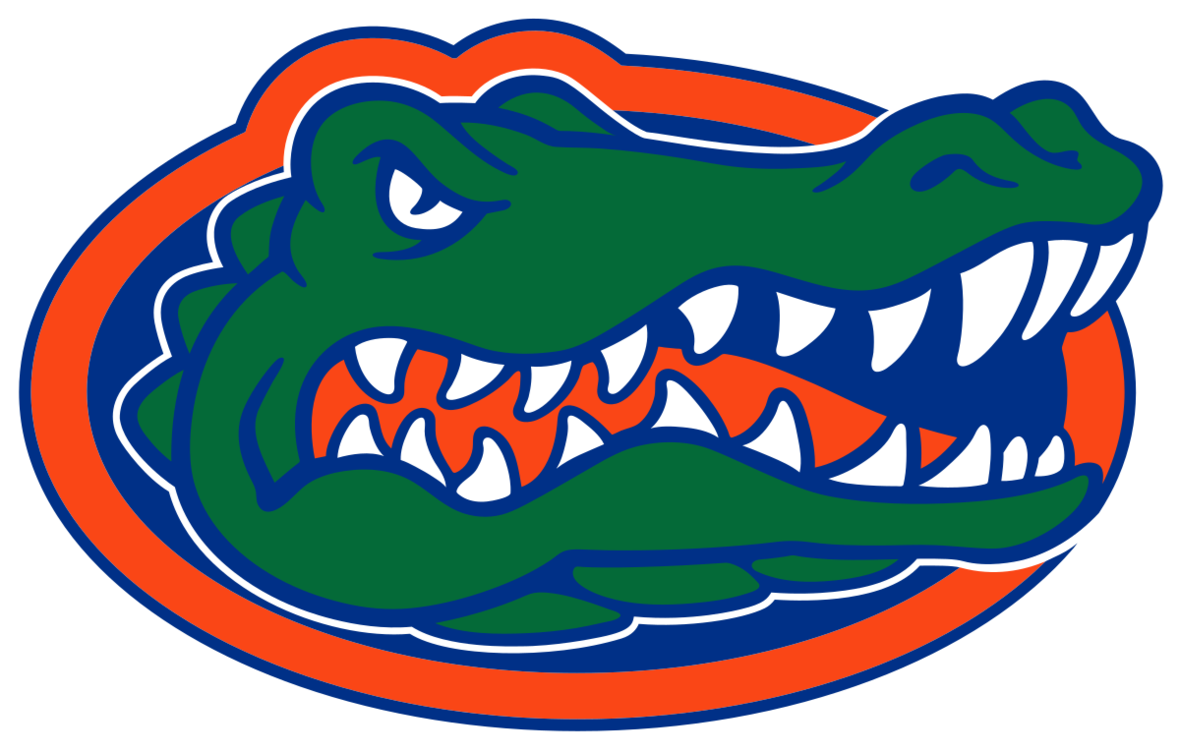/* styles */ Seniors who are applying to UF: If you’re planning to live on campus, submit a housing application and \$25 non-refundable fee as soon as possible. You will receive an email within 24-48 hours of your application submission. Please make sure to submit your deposit. Your Housing Application Date secures your place line for room selection. If you wait until you’re accepted to UF, you will have limited options for campus housing. On campus housing is not guaranteed for incoming students.
 table div table+table+table+table+table+table+table+table+table+table+table+table+table+table+table+table+table+table+table+table+table+table+table+table div table{width:100%;padding:0}table div table+table+table+table+table+table+table+table+table+table+table+table+table+table+table+table+table+table+table+table+table+table+table+table div table img{width:96.23%;padding:0;float:none}table div table+table+table+table+table+table+table+table+table+table+table+table+table+table+table+table+table+table+table+table+table+table+table+table div table td{width:100%;padding:0 1.88% 18px}/* styles */## September Monthly To-Do'sClass of 2020 SEPTEMBER Reminders:

Seniors, set up a formal meeting with your guidance counselor to review your updated transcripts with accurate gpa, class size and rank. Make sure all grade and courses listed are accurate, including any high school credits earned through middle and virtual school.

Many colleges require "recommenders". Confirm the colleges on your list that require letters of recommendation. Email and kindly ask your guidance counselor and two teachers if they are willing to write you a letter of recommendation. Attach your resume. Please respect their time. Make sure to give them a minimum of a three week notice.

September 14th: ACT

September 17th-24th: Late Registration deadline for October 5th, 2019 SAT and Subject Tests.

September 20th: Registration deadline for October 26, 2019 ACT.

September 21st - October 4th: Late Registration deadline for October 26, 2019 SAT.

▪ Make sure all of your Common Application essay drafts are finalized and ready to go.
▪ Continue College visits.
▪ Consider applying Early Decision and/or Early Action.
▪ Begin working on supplemental essays for schools that require them.
 ▪ Make sure all of your Common Application essay drafts are finalized and ready to go.
 ▪ Continue College visits.
 ▪ Consider applying Early Decision and/or Early Action.
 ▪ Begin working on supplemental essays for schools that require them.

Class of 2021 Reminder:

▪ Continue with Test Prep.
▪ Begin work on resumes and college lists!
 ▪ Continue with Test Prep.
 ▪ Begin work on resumes and college lists!
 table div table+table+table+table+table+table+table+table+table+table+table+table+table+table+table+table+table+table+table+table+table+table+table+table+table+table+table+table div table{width:100%;padding:0}table div table+table+table+table+table+table+table+table+table+table+table+table+table+table+table+table+table+table+table+table+table+table+table+table+table+table+table+table div table img{width:96.23%;padding:0;float:none}table div table+table+table+table+table+table+table+table+table+table+table+table+table+table+table+table+table+table+table+table+table+table+table+table+table+table+table+table div table td{width:100%;padding:0 1.88% 18px}/* styles */## Coalition Application SAT Score Send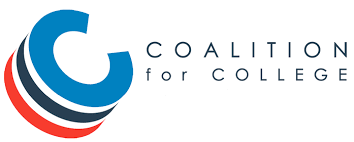/* styles */ SAT Score Send For the first time ever, students can order official SAT scores and submit college applications in the same place: MyCoalition! So far, over 3,000 students have linked their Coalition and College Board accounts! Students no longer have to log into the College Board website separately to send their SAT scores to each college. Plus, if they’ve been awarded a College Board fee waiver, students can send their scores to an unlimited number of schools (even those that aren’t Coalition members) at no cost. You can learn more about our collaboration on the College Board blog.
 table div table+table+table+table+table+table+table+table+table+table+table+table+table+table+table+table+table+table+table+table+table+table+table+table+table+table+table+table+table+table+table+table div table{width:100%;padding:0}table div table+table+table+table+table+table+table+table+table+table+table+table+table+table+table+table+table+table+table+table+table+table+table+table+table+table+table+table+table+table+table+table div table img{width:96.23%;padding:0;float:none}table div table+table+table+table+table+table+table+table+table+table+table+table+table+table+table+table+table+table+table+table+table+table+table+table+table+table+table+table+table+table+table+table div table td{width:100%;padding:0 1.88% 18px}/* styles */## Test Prep tidbitstable div table+table+table+table+table+table+table+table+table+table+table+table+table+table+table+table+table+table+table+table+table+table+table+table+table+table+table+table+table+table+table+table+table+table+table+table div table{width:100%;padding:0}table div table+table+table+table+table+table+table+table+table+table+table+table+table+table+table+table+table+table+table+table+table+table+table+table+table+table+table+table+table+table+table+table+table+table+table+table div table img{width:96.23%;padding:0;float:none}table div table+table+table+table+table+table+table+table+table+table+table+table+table+table+table+table+table+table+table+table+table+table+table+table+table+table+table+table+table+table+table+table+table+table+table+table div table td{width:100%;padding:0 1.88% 18px}/* styles */## Scholarship Opportunities/* styles */ There are thousands of scholarships out there. Below you will find many scholarships, most with general eligibility requirements. My goal is to share some lesser-known scholarships with my readers every Saturday. Click here for DoSomething.org Monthly Scholarships! Click here for October Scholarships! Prudential Spirit of the Community Awards – due November 5, 2019. Students who have given back to their community in a meaningful way, in grades 5-12, and who are legal residents are eligible to apply. Scholarships in the amount of \$1,000 and \$5,000 are available if students continue to advance. Go to: https://spirit.prudential.com/ to apply.
 table div table+table+table+table+table+table+table+table+table+table+table+table+table+table+table+table+table+table+table+table+table+table+table+table+table+table+table+table+table+table+table+table+table+table+table+table+table+table+table+table div table{width:100%;padding:0}table div table+table+table+table+table+table+table+table+table+table+table+table+table+table+table+table+table+table+table+table+table+table+table+table+table+table+table+table+table+table+table+table+table+table+table+table+table+table+table+table div table img{width:96.23%;padding:0;float:none}table div table+table+table+table+table+table+table+table+table+table+table+table+table+table+table+table+table+table+table+table+table+table+table+table+table+table+table+table+table+table+table+table+table+table+table+table+table+table+table+table div table td{width:100%;padding:0 1.88% 18px}/* styles */## SlideRoom for Portfolio Submissions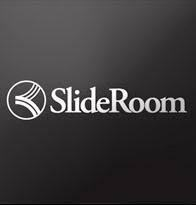/* styles */ SlideRoom is the exclusive partner of The Common Application to receive and review Portfolios from applicants. Each school receives their own branded portal where applicants apply and another portal where reviewers can privately evaluate submitted materials. Click HERE for more information.
 table div table+table+table+table+table+table+table+table+table+table+table+table+table+table+table+table+table+table+table+table+table+table+table+table+table+table+table+table+table+table+table+table+table+table+table+table+table+table+table+table+table+table+table+table div table{width:100%;padding:0}table div table+table+table+table+table+table+table+table+table+table+table+table+table+table+table+table+table+table+table+table+table+table+table+table+table+table+table+table+table+table+table+table+table+table+table+table+table+table+table+table+table+table+table+table div table img{width:96.23%;padding:0;float:none}table div table+table+table+table+table+table+table+table+table+table+table+table+table+table+table+table+table+table+table+table+table+table+table+table+table+table+table+table+table+table+table+table+table+table+table+table+table+table+table+table+table+table+table+table div table td{width:100%;padding:0 1.88% 18px}/* styles */Test prep students, when you are registering for live classes utilizing our Acuity online scheduler, please make sure that you are signing up for the correct class based on your strength test. You should be signing up for either ACT or SAT classes, not the other and not both. This confusion is causing classes to fill up in error. Also, please do not sign up more than once for each individual class as this is also causing classes to fill up in error. We truly appreciate your CAREFUL attention to this matter.

Please register for the ACT at act.org OR SAT at collegeboard.org. Please note there are early registration deadline dates. You can register after the deadline date but a late fee will be required.

 table div table+table+table+table+table+table+table+table+table+table+table+table+table+table+table+table+table+table+table+table+table+table+table+table+table+table+table+table+table+table+table+table+table+table+table+table+table+table+table+table+table+table+table+table+table+table+table div table{width:100%;padding:0}table div table+table+table+table+table+table+table+table+table+table+table+table+table+table+table+table+table+table+table+table+table+table+table+table+table+table+table+table+table+table+table+table+table+table+table+table+table+table+table+table+table+table+table+table+table+table+table div table img{width:96.23%;padding:0;float:none}table div table+table+table+table+table+table+table+table+table+table+table+table+table+table+table+table+table+table+table+table+table+table+table+table+table+table+table+table+table+table+table+table+table+table+table+table+table+table+table+table+table+table+table+table+table+table+table div table td{width:100%;padding:0 1.88% 18px}/* styles */## Scholarships Search Tools

 /* styles */ There are many good scholarship search tools/engines on the internet. Their primary function is to assist you in finding scholarships that match the eligibility criteria you enter for GPA, gender, residency, ethnicity/heritage, religion and area of study, hobbies, interests — some of the most common criteria used. The College Map team recommends the following search engines: Scholarships.com FastWeb Big Future Unigo SallieMae Please make sure to "click" or fill out as much information as possible. Once you've entered your information into the database, the search engine will generate a list of all current scholarships you qualify for. We recommend sorting that list by due date.
 table div table+table+table+table+table+table+table+table+table+table+table+table+table+table+table+table+table+table+table+table+table+table+table+table+table+table+table+table+table+table+table+table+table+table+table+table+table+table+table+table+table+table+table+table+table+table+table+table+table+table div table{width:100%;padding:0}table div table+table+table+table+table+table+table+table+table+table+table+table+table+table+table+table+table+table+table+table+table+table+table+table+table+table+table+table+table+table+table+table+table+table+table+table+table+table+table+table+table+table+table+table+table+table+table+table+table+table div table img{width:96.23%;padding:0;float:none}table div table+table+table+table+table+table+table+table+table+table+table+table+table+table+table+table+table+table+table+table+table+table+table+table+table+table+table+table+table+table+table+table+table+table+table+table+table+table+table+table+table+table+table+table+table+table+table+table+table+table div table td{width:100%;padding:0 1.88% 18px}/* styles */Our private "Your Path" page contains details for upcoming deadlines, events, and important announcements. We encourage you to check it regularly! The password is case-sensitive:
prepare@TCM2018

# Test Prep Schedules

Be sure to register for your test prep classes, available on the Your Path page and the Acuity Scheduler. Registration is mandatory; walk-ins may not be able to be accommodated.

# Suggestions?

Is there something you'd like to see in our newsletter? An event you'd like us to host? A friend you'd like to refer? Let us know by simply replying to this message.

 /* styles */ Kind regards, Your Team at The College Map